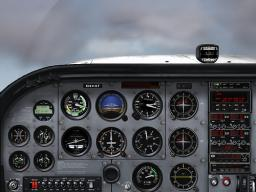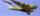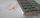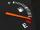# Plane II

A plane flew 50 km on a bearing 63degrees20, and the flew on a bearing 153degrees20 for 140km. Find the distance between the starting point and the ending point.

x =  148.6607 km

### Step-by-step explanation:Did you find an error or inaccuracy? Feel free to write us. Thank you!Tips to related online calculators
Pythagorean theorem is the base for the right triangle calculator.

#### You need to know the following knowledge to solve this word math problem:

We encourage you to watch this tutorial video on this math problem:

## Related math problems and questions:

• BearingA plane flew 50 km on a bearing 63°20' and the flew on a bearing 153°20' for 140km. Find the distance between the starting point and the ending point.A ship travels 84 km on a bearing of 17° and then travels on a bearing of 107° for 135 km. Find the distance of the end of the trip from the starting point, to the nearest kilometer.
• TrianglesFind out whether given sizes of the angles can be interior angles of a triangle: a) 23°10',84°30',72°20' b) 90°,41°33',48°37' c) 14°51',90°,75°49' d) 58°58',59°59',60°3'
• Reverse Pythagorean theoremGiven are lengths of the sides of the triangles. Decide which one is rectangular: Δ ABC: 77 dm, 85 dm, 36 dm ... Δ DEF: 55 dm, 82 dm, 61 dm ... Δ GHI: 24 mm, 25 mm, 7 mm ... Δ JKL: 32 dm, 51 dm, 82 dm ... Δ MNO: 51 dm, 45 dm,
• TableclothsThe restaurant has sixty-two square tablecloths with a side length of 150 cm and 36 rectangular tablecloths with dimensions of 140 cm and 160 cm. A) How many meters of hemming ribbon will be needed if we add 50 cm to each tablecloth? B) The ribbon sale in
• AircraftThe aircraft has in a fuel tank 68 hl of aviation fuel and flight consumes 3.6 liters of fuel. Identify the function, which expresses the dependence of the volume of fuel in tank on the track distance plane flew by. How many hectolites of fuel is still in
• Altitude anglesCities A, B, C lie in one elevation plane. C is 50 km east of B, B is north of A. C is deviated by 50° from A. The plane flies around places A, B, C at the same altitude. When the aircraft is flying around B, its altitude angle to A is 12°. Find the altit
• Above 100%Find 340 percent of the numbers 45 and 55.
• AnglesThe triangle is one outer angle 158°54' and one internal angle 148°. Calculate the other internal angles of a triangle.
• Is right?Is a triangle with sides 51, 56, and 77 right triangle?
• The pondWe can see the pond at an angle 65°37'. Its end points are 155 m and 177 m away from the observer. What is the width of the pond?
• Euclidean distanceCalculate the Euclidean distance between shops A, B, and C, where: A 45 0.05 B 60 0.05 C 52 0.09 The first figure is the weight in grams of bread, and the second figure is the USD price.
• RemaindersIt is given a set of numbers { 170; 244; 299; 333; 351; 391; 423; 644 }. Divide this numbers by number 66 and determine set of remainders. As result write sum of this remainders.
• GasolineFor nearly full tank of 40 liters of gasoline chauffeur paid 54 euros. How many euros it will cost to go 250 km long if the car has an average fuel consumption 7 liters per 100 kilometers?
• Two aircraftTwo planes fly to the airport. At some point, the first airplane is away from the airport 98 km and the second 138 km. The first aircraft flies at an average speed of 420 km/h, the second average speed is 360 km/h, while the tracks of both planes are perp
• Four prismsQuestion No. 1: The prism has the dimensions a = 2.5 cm, b = 100 mm, c = 12 cm. What is its volume? a) 3000 cm2 b) 300 cm2 c) 3000 cm3 d) 300 cm3 Question No.2: The prism base is a rhombus with a side length of 30 cm and a height of 27 cm. The height of t
• Right triangleIt is given a right triangle angle alpha of 90 degrees beta angle of 55 degrees c = 10 cm use Pythagorean theorem to calculate sides a and b